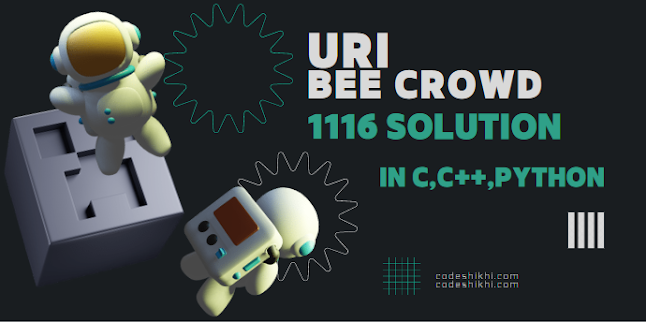# URI / BEE CROWD 1116 - Dividing X by Y - Solution in C,C++,Python | URI - BEECROWD - BEE 1116 Solution in C,C++,Pythonbeecrowd | 1116

# Dividing X by Y

Adapted by Neilor Tonin, URIBrazil

Timelimit: 2

Write a program that read two numbers X and Y and print the result of dividing the X by Y. If it's not possible, print the message "divisao impossivel".

## Input

The input contains an integer number N. This N is the quantity of pairs of integer numbers X and Y read (dividend and divisor).

## Output

For each test case print the result of this division with one digit after the decimal point, or “divisao impossivel” if it isn't possible to perform the calculation.

Obs.: Be carefull. The division between two integers in some languages generates another integer. Use cast:)

 Input Sample Output Sample 33 -2-8 00 8 -1.5divisao impossivel0.0

Demonstration:

This program takes user inputs. Then check the conditions for each test case contains. Finally, print the results.

N.B: Don't copy paste the code as same. Just try to understand it and try yourself.

## URI / BEECROWD Online Judge 1116 Solve in C :

```#include <stdio.h>

int main()
{
int n, i;
float x, y;

scanf("%d", &n);

for(i = 0; i < n; ++i)
{
scanf("%f %f", &x, &y);

if(y == 0)
{
printf("divisao impossivel\n");
}
else
{
printf("%.1f\n", (x/y) );
}
}

return 0;
}
```